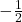## The sum of 8 times a number and 7 equals 3.​

Question

The sum of 8 times a number and 7 equals 3.​

in progress 0
6 months 2021-07-21T02:00:38+00:00 2 Answers 9 views 0Step-by-step explanation:

“The sum of 8 times a number (x) and 7 equals 3.”

Let, “a number” = x

8x + 7 = 3

Isolate the variable, x. Note the equal sign, what you do to one side, you do to the other. Do the opposite of PEMDAS.

PEMDAS is the order of operation, and equals:

Parenthesis

Exponents (& Roots)

Multiplication

Division

Subtraction.

First, subtract 7 from both sides of the equation:

8x + 7 (-7) = 3 (-7)

8x = 3 – 7

8x = -4

Next, divide 8 from both sides of the equation:

(8x)/8 = (-4)/8

x = -4/8

x = -1/2

-1/2 is your number, or -0.5

~